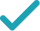Type a math problemSolve for bSteps for Solving Linear Equation
Swap sides so that all variable terms are on the left hand side.
The equation is in standard form.
Divide both sides by .
Dividing by undoes the multiplication by .
Divide by .
Solve for xSteps for Solving Linear Equation
Subtract from both sides.
Subtract from both sides.
Subtract from to get .
Combine all terms containing .
Divide both sides by .
Dividing by undoes the multiplication by .
Divide by .
GraphGraph Both Sides in 2D
Graph in 2DGiving is as easy as 1, 2, 3
Get 1,000 points to donate to a school of your choice when you join Give With Bing
bx-7=8x+5
Swap sides so that all variable terms are on the left hand side.
bx=8x+5+7
bx=8x+12
Add 5 and 7 to get 12.
xb=8x+12
The equation is in standard form.
\frac{xb}{x}=\frac{8x+12}{x}
Divide both sides by x.
b=\frac{8x+12}{x}
Dividing by x undoes the multiplication by x.
b=8+\frac{12}{x}
Divide 8x+12 by x.
8x+5-bx=-7
Subtract bx from both sides.
8x-bx=-7-5
Subtract 5 from both sides.
8x-bx=-12
Subtract 5 from -7 to get -12.
\left(8-b\right)x=-12
Combine all terms containing x.
\frac{\left(8-b\right)x}{8-b}=\frac{-12}{8-b}
Divide both sides by 8-b.
x=\frac{-12}{8-b}
Dividing by 8-b undoes the multiplication by 8-b.
x=-\frac{12}{8-b}
Divide -12 by 8-b.